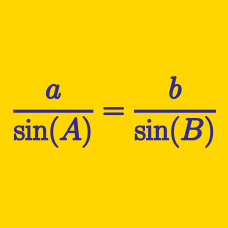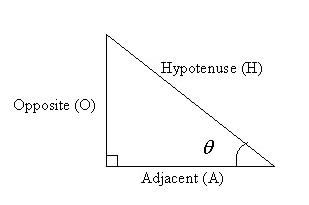Geometry

Lengths in Right TrianglesIn the above right angled triangle, if $\theta=20^\circ$ and the length of the side adjacent to $\theta$ is $98,$ what is the length of the side opposite to $\theta$?In the above right angled triangle, if $\theta=20^\circ$ and the length of the side adjacent to $\theta$ is $21,$ what is the length of the hypotenuse?In the above right angled triangle, if $\theta=30^\circ$ and the length of the side opposite angle $\theta$ is $99$ cm, what is the length of the adjacent side (A) in cm?Given a triangle with one angle of $90^\circ,$ the triangle is called a right angled triangle and the side opposite the right angle is called the hypotenuse. The side opposite the angle $\theta$ in the above diagram is called the opposite side and the remaining side is called the adjacent side. If $\theta=20^\circ$ and the length of the hypotenuse is $81$ cm, what is the length of the opposite side in cm?

Note: The above diagram is not drawn to scale.In the above right angled triangle, if $\theta=20^\circ$ and the length of the side opposite to $\theta$ is $80,$ what is the length of the side adjacent to $\theta$?

×